# Evolute

of a plane curve

The set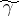of the centres of curvature of the given curve. If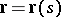(whereis the arc length parameter of) is the equation of, then the equation of its evolute has the form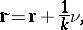whereis the curvature andthe unit normal to. The figures shows the construction of the evolute in three typical cases:

a) if along the entire curve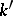has a fixed sign anddoes not vanish;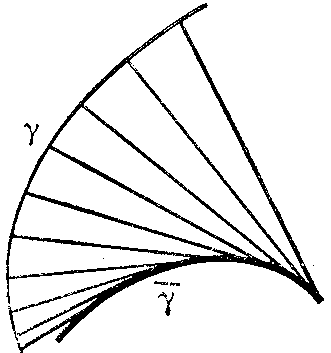Figure: e036670aFigure: e036670b

b) if along the entire curvehas a fixed sign andvanishes for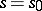; and

c) iffor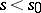;for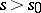;, anddoes not vanish (the point of the evolute corresponding tois a cusp).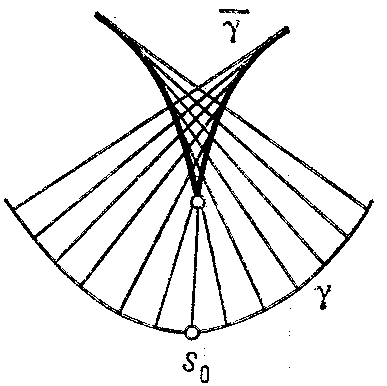Figure: e036670c

The length of the arc of the evolute corresponding to the segment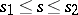ofis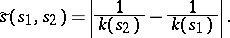The evolute is the envelope of the normals to. The curveis called the evolvent of its evolute (cf. Evolvent of a plane curve).

The evolvent is also called the involute; thus, ifis the evolute of, thenis the involute of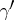, cf. Evolvent of a plane curve.